# Eq with reciprocal

Solve given equation with reciprocal member:

a-6/a+10=4/8

a1 =  0.5944
a2 =  -10.0944

### Step-by-step explanation: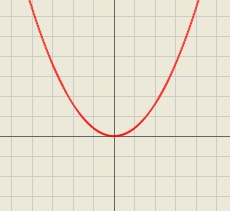Did you find an error or inaccuracy? Feel free to write us. Thank you!Tips to related online calculators

## Related math problems and questions:

• Reciprocal equation 2Solve this equation: x + 5/x - 6 = 4/11
• Reciprocal equationDetermine the root of the equation: 9/x-7/x=1
• Equation - inverseSolve for x: 7: x = 14: 1000
• Reciprocal equation 3Solve reciprocal equation: 1/2 + 2/3=1/x
• Reciprocal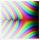What is the reciprocal of the sum of the reciprocals of 7 and 6?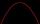Solve pure quadratic equation -5x2 +10 = 0.
• Equation with absSolve this equation with absolute value member: |3(3x-1)|=8
• Simply equationSolve this equation for x: 9x-6(x-1)=5(x+2)-11
• Freedom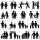In the city are 3/9 of women married for 3/6 men. What proportion of the townspeople is free (not married)? Express as a decimal number.
• Reciprocal value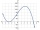How do I calculate a number x that is 9 greater than its reciprocal (1/x)?
• EQ2Solve quadratic equation: 2x²- 2(x-7)²+5x=0
• Reciprocal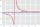It is true (prove it) that if a> b> 0: ?
• Reciprocal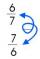Calculate the reciprocal numbers for the given real numbers.
• Simple equation 6Solve equation with one variable: X/2+X/3+X/4=X+4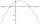It is given a quadratic function y = -4x2+5x+c with unknown coefficient c. Determine the smallest integer c for which the graph of f intersects the x-axis at two different points.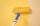Redecoration school had scheduled for four crew to 10 days. After two days a single worker ill. For how many days the school was painted when remain workers working with the same tempo?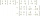Explain why the reciprocal of - 1 1/17 is the multiplicative inverse of - 17/18.